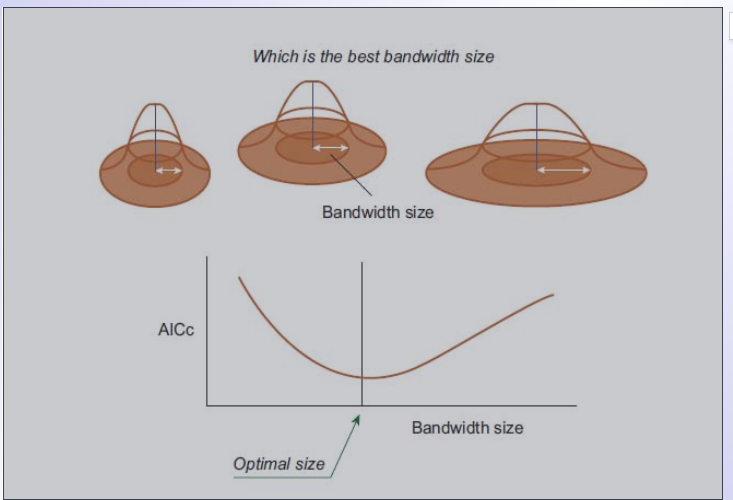# 空间计量：地理加权归回模型-(GWR)-参数估计

Stata 连享会   主页 || 视频 || 推文

Email: chenfengscsfdx@163.com

## 3. 常用的核函数

### 3.2. Bi-square kernel function

$$w_i(u_j,v_j)=K_h(d_{ij})=\left\{ \begin{array}{rcl} \left[1-\left(\frac{d_{ij}}{h}\right)^2\right]^2, & \left|d_{ij}\right|h. \end{array}\right.$$

### 3.3. K-nearest neighbor kernel function

$$w_i(u_j,v_j)=K_h(d_{ij})=\left\{ \begin{array}{rcl} \left[1-\left(\frac{d_{ij}}{d_{ik}}\right)^2\right]^2, & \left|d_{ij}\right|d_{ik}. \end{array}\right.$$

## 4. 窗宽h的选择准则

### 4.3. AICc信息准则(Corrected Akaike information criterion (AICc))

$\mathrm{A}\mathrm{I}\mathrm{C}\mathrm{c}$ 准则选择最优窗宽如下所示：注：模拟实验以及经验表明，$\mathrm{C}\mathrm{V}$ 和 $\mathrm{G}\mathrm{C}\mathrm{V}$ 准则一般会趋于确定一个稍微偏小的窗宽${h}_{0}$，而较小的窗宽会使得回归函数的估计值偏差减小，但是方差会增大。因此，会出现过拟合现象。但是对于 $\mathrm{A}\mathrm{I}\mathrm{C}\mathrm{c}$ 在很多情况下可以较好的克服过拟合现象，即趋于确定一个更合理的窗宽。

## 4. 在 R 软件运行地理加权回归模型

# 1. 加载 Rpacakge：
library("GWmodel")

# 2. 加载数据
data(DubVoter)

# 3. 选择最优窗宽
Dub<cbind(Dub.voter\$DiffAdd,Dub.voter\$LARent,Dub.voter\$SC1,Dub.voter\$Unempl,Dub.voter\$LowEduc,Dub.voter\$Age18_24,Dub.voter\$Age25_44,Dub.voter\$Age45_64,Dub.voter\$GenEl2004) DubCoord<-cbind(Dub.voter\$X,Dub.voter\$Y) %地理位置 DIS<-gw.dist(dp.locat=DubCoord)% 计算距离矩阵 bw1<bw.gwr(GenEl2004~DiffAdd+LARent+SC1+Unempl+LowEduc+Age18_24+Age25_44+Age45_64, approach="AICc",adaptive=TRUE, data=Dub.voter, kernel = "bisquare",dMat=DIS) %选择bi-square函数作为核函数，使用AICc准则选择最优窗宽 # 4. 拟合GWR模型 gwr.res1<gwr.basic(GenEl2004~DiffAdd+LARent+SC1+Unempl+LowEduc+Age18_24+Age25_44+Age45_64, data=Dub.voter, bw=bw1,adaptive=TRUE,kernel = "bisquare", dMat=DIS) # 5. 将局部估计值画在对应的地图上面 library("RColorBrewer") Mcolor<-1;mypalette <- colorRampPalette(brewer.pal(9, "Greys"))(200) map.na=list("SpatialPolygonsRescale", layout.north.arrow(), offset=c(329000, 261500), scale=4000,col=1) map.scale.1=list("SpatialPolygonsRescale",layout.scale.bar(), offset=c(326500, 217000), scale=5000,col=1, fill=c("transparent","black")) map.scale.2=list("sp.text",c(326500, 217900),"0",cex=0.9,col=1) map.scale.3=list("sp.text",c(331500, 217900),"5km",cex=0.9,col=1) map.layout<-list(map.na,map.scale.1,map.scale.2,map.scale.3) mypalette.9<-brewer.pal(9,"Greys") spplot(gwr.res1$SDF, "LowEduc",key.space="right",
col.regions=mypalette.6, at=c(-8,-6,-4,-2,0,2,4),
sp.layout=map.layout)


## 5. 参考文献

• Brunsdon, C. E, Fotheringham, A. S. and Charlton, M. E., 1999. Some notes on parametric significance test for geographically weighted regression. Journal of Regional Science, 39 (3): 497–524. [PDF]
• 梅长林, 王宁. 近代回归分析方法 [M]: 北京：科学出版社, 2012.
• Lu, B. B., Harris, P., Charlton, M. and Brunsdon, C., 2014. The GWmodel R package: further topics for exploring spatial heterogeneity using geographically weighted models. Geo-spatial Information Science, 17 (2): 85–101. [PDF]

## 相关课程

http://lianxh.duanshu.com

### 课程一览

Stata数据清洗 游万海 直播, 2 小时，已上线

Note: 部分课程的资料，PPT 等可以前往 连享会-直播课 主页查看，下载。

#### 关于我们

• Stata连享会 由中山大学连玉君老师团队创办，定期分享实证分析经验。直播间 有很多视频课程，可以随时观看。
• 连享会-主页知乎专栏，300+ 推文，实证分析不再抓狂。
• 公众号推文分类： 计量专题 | 分类推文 | 资源工具。推文分成 内生性 | 空间计量 | 时序面板 | 结果输出 | 交乘调节 五类，主流方法介绍一目了然：DID, RDD, IV, GMM, FE, Probit 等。
• 公众号关键词搜索/回复 功能已经上线。大家可以在公众号左下角点击键盘图标，输入简要关键词，以便快速呈现历史推文，获取工具软件和数据下载。常见关键词：课程, 直播, 视频, 客服, 模型设定, 研究设计, stata, plus, 绘图, 编程, 面板, 论文重现, 可视化, RDD, DID, PSM, 合成控制法